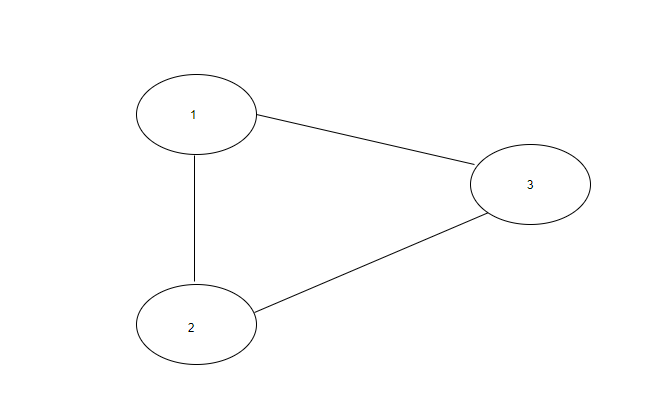New update is available. Click here to update.

# Cycle Detection In Undirected Graph

Last Updated: 1 Jan, 2021
Difficulty: Moderate

## PROBLEM STATEMENT

#### Example :

``````In the below graph, there exists a cycle between vertex 1, 2 and 3.
``````#### Note:

``````1. There are no parallel edges between two vertices.

2. There are no self-loops(an edge connecting the vertex to itself) in the graph.

3. The graph can be disconnected.
``````

#### For Example :

``````Input: N = 3 , Edges =  [[1, 2], [2, 3], [1, 3]].
Output: Yes

Explanation : There are a total of 3 vertices in the graph. There is an edge between vertex 1 and 2, vertex 2 and 3 and vertex 1 and 3. So, there exists a cycle in the graph.
``````
##### Input Format:
``````The first line of input contains an integer 'T' which denotes the number of test cases or queries to be run. Then the test cases follow.

The first line of each test case contains two single space-separated integers ‘N’ and ‘M’ representing the total number of vertices and edges, respectively.

The next ‘M’ lines contain two single space-separated integers representing an edge of the graph.
``````
##### Output Format:
``````For each test case, the only line of output will return “Yes” if there exists a cycle in the graph. Else print “No”.
``````
##### Note:
``````You are not required to print the expected output, it has already been taken care of. Just implement the function.
``````
##### Constraints:
``````1 <= T <= 10
1 <= N <= 5000
0 <= M <= min(5000, (N * (N - 1)) / 2)
1 <= edges[i] <= N
1 <= edges[i] <= N

Time Limit: 1 sec
``````## Approach 1

There is a cycle in the graph only if there is a back edge (back edge is an edge that connects a vertex to another vertex that is discovered before it's parent) present in the graph. To detect a back edge, we will keep track of vertices that have been already visited. If we reach a vertex that is already visited and is not the parent vertex of the current vertex, then there is a cycle in the graph.

Here is the complete algorithm:

1. We create a graph using the ‘EDGES’ array and initialise an array ‘VISITED’ to keep track of the visited vertices.
2. We iterate over all vertices of the graph and if the vertex is unvisited, we call the ‘IS_CYCLEfunction from that vertex. The ‘IS_CYCLE’ function works as follows:
1. Mark the current vertex true in the ‘VISITED’ array.
2. Find all the adjacent vertices of the current vertex.
1. If an adjacent vertex is not visited
1. Recursively call the ‘IS_CYCLE’ function for the adjacent vertex.
2. If the recursive function returns true, then return true.
2. Else if the adjacent vertex is already visited and is not the parent vertex of the current vertex.
1. Return true.
3. Finally, return false.
3. If the ‘IS_CYLCE’ function returns true, then return “Yes”. Else return “No”.